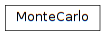# 6.4. pyopus.design.mc — Monte Carlo analysis¶Monte Carlo analysis (PyOPUS subsystem name: MC)

Estimates the yield taking into account operating and statistical parameters. If at least one operating parameter is specified the yield of the worst performance across the statistical parameters is computed.

The bounds on the performance are specified with the lower and the upper entry of the measurement description dictionary.

Statistical parameters are assumed to be independent with zero mean and variance one.

Can also be used for estimating the worst case performance.

class pyopus.design.mc.MonteCarlo(heads, analyses, measures, statParamDesc, opParamDesc, fixedParams={}, variables={}, debug=0, nSamples=1000, storeStatSamples=False, storeOpParams=False, storeWcEvals=False, wcOptions={}, spawnerLevel=1)

See PerformanceEvaluator for details on heads, analyses, measures, and variables.

Statistical parameters and operating parameters are given by dictionaries statParamDesc and opParamDesc. These dictionaries have parameter name for key and parameter property dictionary with lo and hi specifying the lower and the upper bound. The nominal value is given by the init member.

Fixed parameters are given by fixedParams - a dictionary with parameter name for key and parameter value for value. Alternatively the value can be a dictionary in which case the init member specifies the parameter value.

If fixedParams is a list the members of this list must be dictionaries describing parameters. The set of fixed parameters is obtained by merging the information from these dictionaries.

Setting debug to a value greater than 0 turns on debug messages. The verbosity is proportional to the specified number.

Setting debug to a value greater than 0 turns on debug messages. The verbosity is proportional to the specified number.

nSamples is the number of Monte Carlo samples.

By setting storeStatSamples to True storing of the vector of statistical parameters in the statSamples member is enabled. The first index is the sample index, while the second index is the parameter index. Parameters are ordered according to the statNames list.

storeOpParams turns on storing of the worst operating parameters in the results structure.

storeWcEvals turns on storing the number of performance measure evaluations in the results structure.

wcOptions are the worst case analysis options passed to the WorstCase object performing the worst case analysis in the space of the operating parameters.

This is a callable object with at most one argument. If given the argument is a list of entries. Every entry is

• a tuple of the form (name,type), where name is the measure name and type is lower or upper
• a string specifying the measure name. In this case the type of the performance costraint for which the Monte-Carlo analysis is performed is given by the presence of the lower and the upper entries in the performance measure’s description. A separate yield is computed for lower and upper.

If no argument is specified, all yields corresponding to lower/upper bounds of all performance measures are computed.

Results are stored in a results dictionary with pairs of the form (name,type) for key. Values are a dictionaries with the following members:

• samples - a vector of peformance measure values
• op - 2-dimensional array with worst operating parameter values. The first index is the sample index. The second index is the parameter index. The parameters are ordered according to the opNames member of the object. This member is available if storeOpParams* is set to ``True.
• evals - array with the number of performance measure evaluations corresponding to individual samples. Available if storeWcEvals is set to True.
• feasible - number of feasible samples (samples satisfying the performance constraint).
• failed - number of failed samples for which the evaluation failed.
• yield - yield obtained by dividing teh number of feasible samples with nSamples

The results are stored in the results member. The number of analyses performed during the last call to the MonteCarlo object are stored in the analysisCount member.

A call to an object of this class returns a tuple holding the results structure and the analysisCount dictionary.

The totalCheck member holds an array of booleans. Every entry corresponds to one evaluated sample. True means that a sample satisfies all performance constraints.

The totalYield member holds the total yield obtained by the analysis (share of the samples that satisfy all performance constraints).

The statSamples member is a 2-dimensional array holding the stored statistical parameter samples. The first index is the sample index while the second index is the statistical parameter index. The ordering of statistical parameters is given by the statNames member. This member is available if storeStatSamples is set to True.

Setting spawnerLevel to a value not bigger than 1 distributes the evaluations across available computing nodes.

formatResults(nMeasureName=10, nResult=14, nPrec=5, nSamplePrec=4)

Formats the results as a string.

nMeasureName specifies the formatting width for the performance measure name.

nResult and nPrec specify the formatting width and the number of significant digits for the performance measure values.

nPrec specifies the number of significant digits for the results.

nSamplePrec specifies the formatting width for the sample count.

#### Previous topic

6.3. pyopus.design.wcd — Worst case distance computation

#### Next topic

6.5. pyopus.design.cbd — Sizing across corners